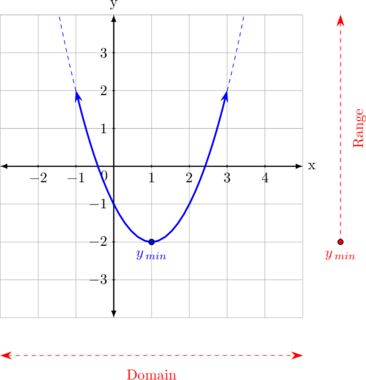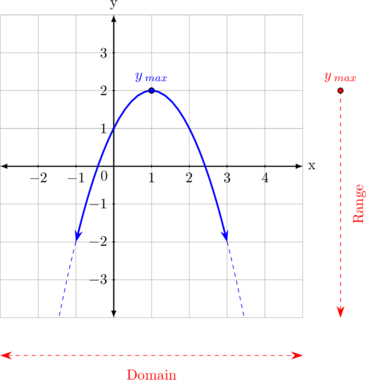# Domain and Range

Quadratic function can be written as:

• $$f : x \rightarrow ax^2 + bx + c$$
• $$f(x) = ax^2 + bx + c$$
• $$y = ax^2 + bx + c$$

Quadratic function can be drawn in Cartesian diagram as a parabolic like below:

Concave-up parabolic curveThe parabolic curve can be extended to the top left and the top right, hence the value of x can be any number.

The curve has minimum value of y, but it does not have maximum value (the curve can go up to infinite), hence the value of y must be greater or equal than $$y_{min}$$.

Concave-down parabolic curveThe parabolic curve can be extended to the bottom left and the bottom right, hence the value of y can be any number.

The curve has maximum value of y, but it does not have minimum value (the curve can go down to infinite), hence the value of y must be less or equal than $$y_{max}$$.

###### Domain and Range of Quadratic Function

Domain

$$x \in R$$ or can be written as $$- \sim \: < x < +\sim$$

Range for concave-up parabolic curve

$$y \geq y_{min}$$

or can be written as

$$y_{min} \leq y < + \sim$$

Range for concave-down parabolic curve

$$y \leq y_{max}$$

or can be written as

$$- \sim \: < y \leq y_{max}$$

Determine the maximum or minimum value of y of the function $$y = ax^2 + bx + c$$

First, find the x value for maximum/minimum point, $$x_{min} = - \dfrac ba$$

Then substitute the value of $$x_{min}$$ into $$y = ax^2 + bx + c$$ to find the value of y.